EN | ES | DE | NL | RU

## Orbitals and Placing Electrons to Orbitals with Examples

Orbitals and Placing Electrons to Orbitals with Examples

When external energy is given to atoms, some of them change their energy levels. We call this state of atom as; exited state. For example, following electron configurations belong to 8O, one of them is ground state and other one is exited state.

1s22s22p4 : "ground state"

1s22s22p33s1 : "exited state"

Elements are in ground states most of the time. When we solve examples you should always take them in ground state.

Showing Electrons in Orbitals (sub shells)

We show orbitals with following shape;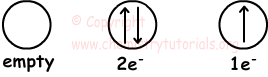Capacity of each orbital is two electrons. We can also show electrons in orbitals as follows ;There are some rules for placing electrons in orbitals. For example, electrons must be placed orbitals having same energy level one by one. If orbitals are not filled with electrons, you can not pass another energy level. Look at the following figure that shows number of orbitals in each sub level s, p, d, f;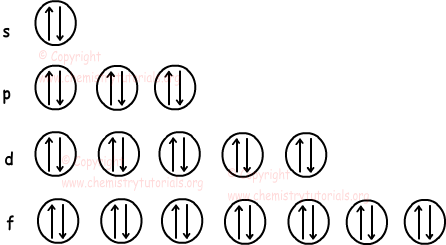Examine following examples to understand placing electrons in orbitals.

1. 5B: 1s22s22p1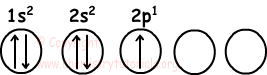2. 6C: 1s22s22p2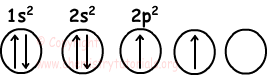3. 7N: 1s22s22p3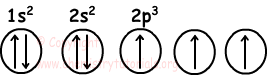4. 8O: 1s22s22p4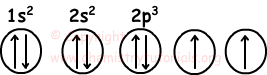Spherical Symmetry

Spherical symmetry makes atom more stable. Half filled or filled orbitals show us spherical symmetry.

s : 1e- or 2e-

p : 3e- or 6e-

d : 5e- or 10e-

f: 7e- or 14e-

Orbitals s, p, d, f have given electrons above, has spherical symmetry.

7N=1s22s22p3 p orbital has 3 electrons, thus N has spherical symmetry.

8O=1s22s22p4 p orbital has 4 electrons, thus O has NO spherical symmetry.

Electron Configurations of Ions

If  electron is bound to neutral atom, atom becomes negatively charged ion. We calculate total number of electrons and make electron configuration.

Example: Write electron configuration of  9F-.

F ion has 9+1=10 electrons.

9F-=1s22s22p6

If electron gives electron it becomes positively charged ion.

Example: Write electron configuration of  14Si+. 14Si+2, 14Si+3 .

14Si+ has 14-1=13 electrons

1s22s22p63s23p1

14Si+2 has 14-2=12 electrons

1s22s22p63s2

14Si+3 has 14-3=11 electrons

1s22s22p63s1

Atomic Structure Exams and  Problem Solutions

The Original Author: